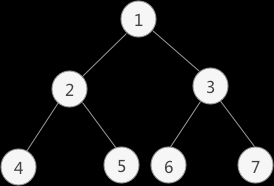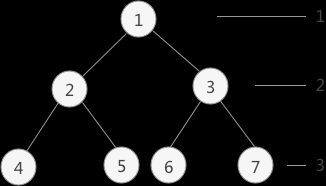# 二叉树的四种遍历方式(递归和非递归双重实现)

08/05 15:51

``````typedef struct BiTNode{
TElemType data;//数据域
struct BiTNode *lchild,*rchild;//左右孩子指针
}BiTNode,*BiTree;
``````

# 1.二叉树的先序遍历

#### 1.1二叉树先序遍历的实现思想是：

1. 访问根节点；
2. 访问当前节点的左子树；
3. 若当前节点无左子树，则访问当前节点的右子树；#### 1.2先序遍历递归实现

``````void PreOrderTraverse(BiTree T){
if (T) {
printf("%d ",T->data);
PreOrderTraverse(T->lchild);//访问该结点的左孩子
PreOrderTraverse(T->rchild);//访问该结点的右孩子
}
//如果结点为空，返回上一层
return;
}
``````

#### 1.3先序遍历非递归实现

``````//先序遍历非递归算法
int top=-1;//top变量时刻表示栈顶元素所在位置
//前序遍历使用的进栈函数
void push(BiTNode** a,BiTNode* elem){
a[++top]=elem;
}
//弹栈函数
void pop( ){
if (top==-1) {
return ;
}
top--;
}
//模拟操作结点元素的函数，输出结点本身的数值
void displayElem(BiTNode* elem){
printf("%d ",elem->data);
}
//拿到栈顶元素
BiTNode* getTop(BiTNode**a){
return a[top];
}
void PreOrderTraverse(BiTree Tree){
BiTNode* a;//定义一个顺序栈
BiTNode * p;//临时指针
push(a, Tree);//根结点进栈
while (top!=-1) {
p=getTop(a);//取栈顶元素
pop();//弹栈
while (p) {
displayElem(p);//调用结点的操作函数
//如果该结点有右孩子，右孩子进栈
if (p->rchild) {
push(a,p->rchild);
}
p=p->lchild;//一直指向根结点最后一个左孩子
}
}
}
``````

# 2.二叉树的中序遍历

#### 2.1二叉树中序遍历思想

1. 访问当前节点的左子树；
2. 访问根节点；
3. 访问当前节点的右子树#### 2.2中序遍历递归实现

``````
//中序遍历
void INOrderTraverse(BiTree T){
if (T) {
INOrderTraverse(T->lchild);//遍历左孩子
printf("%d ",T->data);
INOrderTraverse(T->rchild);//遍历右孩子
}
//如果结点为空，返回上一层
return;
}
``````

#### 2.3中序遍历非递归实现

``````int top=-1;//top变量时刻表示栈顶元素所在位置
//前序和中序遍历使用的进栈函数
void push(BiTNode** a,BiTNode* elem){
a[++top]=elem;
}
//弹栈函数
void pop( ){
if (top==-1) {
return ;
}
top--;
}
//模拟操作结点元素的函数，输出结点本身的数值
void displayElem(BiTNode* elem){
printf("%d ",elem->data);
}
//拿到栈顶元素
BiTNode* getTop(BiTNode**a){
return a[top];
}
//中序遍历非递归算法
void InOrderTraverse1(BiTree Tree){
BiTNode* a;//定义一个顺序栈
BiTNode * p;//临时指针
push(a, Tree);//根结点进栈
while (top!=-1) {//top!=-1说明栈内不为空，程序继续运行
while ((p=getTop(a)) &&p){//取栈顶元素，且不能为NULL
push(a, p->lchild);//将该结点的左孩子进栈，如果没有左孩子，NULL进栈
}
pop();//跳出循环，栈顶元素肯定为NULL，将NULL弹栈
if (top!=-1) {
p=getTop(a);//取栈顶元素
pop();//栈顶元素弹栈
displayElem(p);
push(a, p->rchild);//将p指向的结点的右孩子进栈
}
}
}
``````

``````//中序遍历实现的另一种方法
void InOrderTraverse2(BiTree Tree){
BiTNode* a;//定义一个顺序栈
BiTNode * p;//临时指针
p=Tree;
//当p为NULL或者栈为空时，表明树遍历完成
while (p || top!=-1) {
//如果p不为NULL，将其压栈并遍历其左子树
if (p) {
push(a, p);
p=p->lchild;
}
//如果p==NULL，表明左子树遍历完成，需要遍历上一层结点的右子树
else{
p=getTop(a);
pop();
displayElem(p);
p=p->rchild;
}
}
}
``````

# 3.二叉树的后序遍历

#### 3.1后序遍历思想#### 3.2后序遍历的递归实现

``````void PostOrderTraverse(BiTree T){
if (T) {
PostOrderTraverse(T->lchild);//遍历左孩子
PostOrderTraverse(T->rchild);//遍历右孩子
printf("%d ",T->data);
}
//如果结点为空，返回上一层
return;
}
``````

#### 3.2后序遍历的非递归实现

``````int top=-1;//top变量时刻表示栈顶元素所在位置
//弹栈函数
void pop( ){
if (top==-1) {
return ;
}
top--;
}
//模拟操作结点元素的函数，输出结点本身的数值
void displayElem(BiTNode* elem){
printf("%d ",elem->data);
}
//后序遍历非递归算法
typedef struct SNode{
BiTree p;
int tag;
}SNode;
//后序遍历使用的进栈函数
void postpush(SNode *a,SNode sdata){
a[++top]=sdata;
}
//后序遍历函数
void PostOrderTraverse(BiTree Tree){
SNode a;//定义一个顺序栈
BiTNode * p;//临时指针
int tag;
SNode sdata;
p=Tree;
while (p||top!=-1) {
while (p) {
//为该结点入栈做准备
sdata.p=p;
sdata.tag=0;//由于遍历是左孩子，设置标志位为0
postpush(a, sdata);//压栈
p=p->lchild;//以该结点为根结点，遍历左孩子
}
sdata=a[top];//取栈顶元素
pop();//栈顶元素弹栈
p=sdata.p;
tag=sdata.tag;
//如果tag==0，说明该结点还没有遍历它的右孩子
if (tag==0) {
sdata.p=p;
sdata.tag=1;
postpush(a, sdata);//更改该结点的标志位，重新压栈
p=p->rchild;//以该结点的右孩子为根结点，重复循环
}
//如果取出来的栈顶元素的tag==1，说明此结点左右子树都遍历完了，可以调用操作函数了
else{
displayElem(p);
p=NULL;
}
}
}
``````

# 4.二叉树的层序遍历``````#include <stdio.h>
#define TElemType int
//初始化队头和队尾指针开始时都为0
int front=0,rear=0;
typedef struct BiTNode{
TElemType data;//数据域
struct BiTNode *lchild,*rchild;//左右孩子指针
}BiTNode,*BiTree;
void CreateBiTree(BiTree *T){
*T=(BiTNode*)malloc(sizeof(BiTNode));
(*T)->data=1;
(*T)->lchild=(BiTNode*)malloc(sizeof(BiTNode));
(*T)->rchild=(BiTNode*)malloc(sizeof(BiTNode));

(*T)->lchild->data=2;
(*T)->lchild->lchild=(BiTNode*)malloc(sizeof(BiTNode));
(*T)->lchild->rchild=(BiTNode*)malloc(sizeof(BiTNode));
(*T)->lchild->rchild->data=5;
(*T)->lchild->rchild->lchild=NULL;
(*T)->lchild->rchild->rchild=NULL;

(*T)->rchild->data=3;
(*T)->rchild->lchild=(BiTNode*)malloc(sizeof(BiTNode));
(*T)->rchild->lchild->data=6;
(*T)->rchild->lchild->lchild=NULL;
(*T)->rchild->lchild->rchild=NULL;

(*T)->rchild->rchild=(BiTNode*)malloc(sizeof(BiTNode));
(*T)->rchild->rchild->data=7;
(*T)->rchild->rchild->lchild=NULL;
(*T)->rchild->rchild->rchild=NULL;

(*T)->lchild->lchild->data=4;
(*T)->lchild->lchild->lchild=NULL;
(*T)->lchild->lchild->rchild=NULL;
}
//入队函数
void EnQueue(BiTree *a,BiTree node){
a[rear++]=node;
}
//出队函数
BiTNode* DeQueue(BiTNode** a){
return a[front++];
}
//输出函数
void displayNode(BiTree node){
printf("%d ",node->data);
}
int main() {
BiTree tree;
//初始化二叉树
CreateBiTree(&tree);
BiTNode * p;
//采用顺序队列，初始化创建队列数组
BiTree a;
//根结点入队
EnQueue(a, tree);
//当队头和队尾相等时，表示队列为空
while(front<rear) {
//队头结点出队
p=DeQueue(a);
displayNode(p);
//将队头结点的左右孩子依次入队
if (p->lchild!=NULL) {
EnQueue(a, p->lchild);
}
if (p->rchild!=NULL) {
EnQueue(a, p->rchild);
}
}
return 0;
}
``````

0
0 收藏

### 作者的其它热门文章0 评论
0 收藏
0